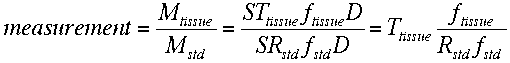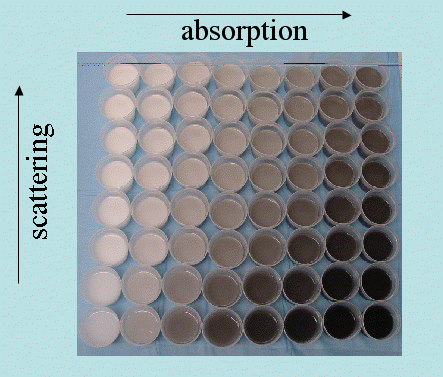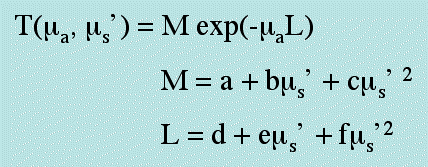# 5. Calibration.

Each test spectrum was normalized by a standard spectrum made of a 99% reflector from a distance of a fixed distance of about 15 cm. The ratio of test/standard constituted a measurement. Both tissue samples and calibration gels (see below) were acquired as such normalized measurement. The cancellation of any day-to-day variation in wavelength dependent light source strength (S) and detector sensitivity (D, in lab units) was achieved. The fraction of transport Ttissue collected by the fiber (ftissue) and the transport and fractional collection in the standard (Rstd and fstd) remained as experimental constants.Although we could approximately model the fiber spectrometer using Monte Carlo, the small details of construction required an experimental calibration. An array of gel phantoms was prepared using polyacrylamide gels with added scatterer (Intralipid TM lipid droplets) and added absorber (India ink).For each of the 64 gels, a full white light reflectance spectrum was taken yielding 220 values. The 14080 data were binned into values at intervals of absorption coefficient, ua [cm-1], and reduced scattering coefficient, us' [cm-1]. Then for each bin of scattering, all the data for various absorption were plotted as M exp(- uaL) where L is an apparent pathlength for photons. The values of M and L were established for each bin of scattering. Finally, the variation of M and L as functions of us' was determined. The result is summarized as follows:The behavior of both types of probes was able to be characterized by the above simple expression. The scattering scales the magnitude (M) of transport and the apparent pathlength (L) of photons. The absorption attenuates the transport over the pathlength L(us'). The values of a, b, c, d, e and f characterizes the scattering dependence of M(us') and L(us'). The terms c and f were very small and to a first approximation the functions M and L were linear with respect to us'.

Hence, a simple algorithm mimiced the transport function for a particular probe. Experimental calibration was able to specify the calibration for each probe, but both probes followed this general pattern of behavior.